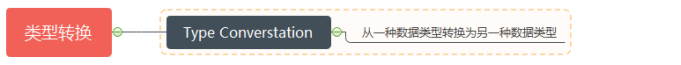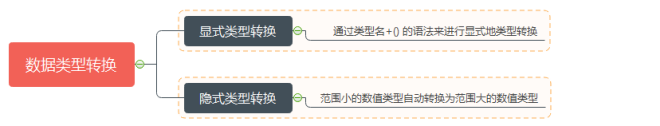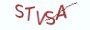Python基础教程

# 8.3.1 理解数据类型转换`需对生活抱持美好的期待，虽然它充满着波折和磨难，勿需遗忘你本来的样子，要成为你想变成的样子`

# 8.3.2 显式类型转换

```# 据说单身狗荷包里会有11块1毛1分钱, 还经常舍不得花
money = 11.11
money = int(money)
# 据说有对象的人，从相识到结婚，平均会花费￥201314元
money = -201314
money = float(money)```

```(1) 在代码实例中定义了一个浮点类型的变量money,然后通过int(money)的形式将浮点类型显式地转换为整型。
(1) 将整型转换为浮点类型时，数字后面会多了一个小数点。例如将201314转换为浮点类型，则转换后的值为201314.0```

```# 1.字符串类型转换为数值类型
number = "  201314"
# 转换为十进制整数，base表示转换的基数
number = int(number, base=10)
number = "  1010"
# 转换为十进制整数，base表示转换的基数
number = int(number, base=2)
# 转换为浮点类型
number = "  201314"
number = float(number)
# 2.数值类型转换为字符串类型
number = str(number)```

(1) 将字符串类型转换为数值类型时，直接通过int()或float()将字符串类型显式转换为数值类型

(2) 对于整数，可以在int()的显式转换中，通过参数base来指定转换的基数，字符串中的数字表示十进制时，传递的参数为10。

字符串中的数字表示二进制时，传递的参数为2。字符串中的数字表示十六进制时，传递的参数为16。

(3) 通过str()将数值类型转换为字符串类型

```number = "  -20.1314  "
# 类型转换成功
number = int(number)

number = "  -ABC  "
# 类型转换成功
number = int(number, base=16)
number = "20.1314    748"
# 转换为数值类型时抛出异常
number = int(number)
number = "20.1314  \n"
# 转换为数值类型时抛出异常
number = float(number)```

```(1) 字符串"20.1314    748"不是连续的数字
(2) 字符串"20.1314  \n"包含非法的字符```

# 8.3.3 隐式类型转换

`布尔类型，整型，浮点类型`

`布尔类型 < 整型 < 浮点类型`

```# 布尔类型自动转换为范围更大的整型
# True转换后的整型值为1，False转换后的整型值为0
value = 1+ True
# value的值为2

# 布尔类型自动转换为范围更大的浮点类型
# True转换后的浮点值为1.0，False转换后的浮点值为0.0
value = 1.0+ True
# value的值为2.0

# 范围小的数据类型自动转换为范围更大的数据类型
value = 1+1.0+ True
# value的值为3.0```

`(1) 范围小的数据类型自动转换为范围大的数据类型(2) 在表达式1+1.0+True中，按左结合性先计算1+1.0,1是整型，1.0是浮点类型，整型1自动转换为浮点类型1.0,1+1.0的结果为2.0。同理2.0+True等价于2.0+1.0,值为3.0`# 8.3.4 其它类型与布尔类型的转换

`在条件控制结构和循环控制结构中，会将表达式的值转换为逻辑值，如果为真值就执行相应的代码。`

# 8.3.5 本节知识要点

`(1) 数据类型转换是指将一种数据类型转换为另一种数据类型(2) Python中的数据类型转换有显式类型转换和隐式类型转换 (3) 整型和浮点类型的0值，空字符串，None类型等转换后的布尔类型为False`

# 8.3.6 高薪就业班

`(1) Python后端工程师高薪就业班，月薪11K-18K，免费领取课程大纲(2) Python爬虫工程师高薪就业班，年薪十五万，免费领取课程大纲(3) Java后端开发工程师高薪就业班，月薪11K-20K, 免费领取课程大纲(4) Python大数据分析，量化投资就业班，月薪12K-25K,免费领取课程大纲`## 注册账号